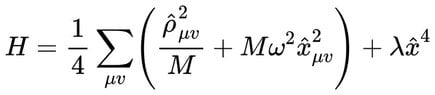# What is the oscillator model in a generalized Snyder scheme？

Zhiping Lai
Homework Statement:
Relevant Equations:
$$H= \frac{1}{4} \sum_{\mu v}\left(\frac{\hat{\rho}_{\mu v}^{2}}{M}+M \omega^{2} \hat{x}_{\mu v}^{2}\right)+\lambda \hat{x}^{4},$$
What is the oscillator model in a generalized Snyder scheme？How to derive the formula？

#### Attachments

•formula.jpg
11.2 KB · Views: 7